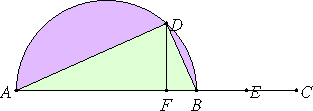# Proposition 35

To find two straight lines incommensurable in square which make the sum of the squares on them medial and the rectangle contained by them medial and moreover incommensurable with the sum of the squares on them.

Set out two medial straight lines AB and BC commensurable in square only, containing a medial rectangle, such that the square on AB is greater than the square on BC by the square on a straight line incommensurable with AB. Describe the semicircle ADB on AB, and make the rest of the construction as above.

Since AF is incommensurable in length with FB, therefore AD is also incommensurable in square with DB.Since the square on AB is medial, therefore the sum of the squares on AD and DB is also medial.

X.32,Cor.

Since the rectangle AF by FB equals the square on each of the straight lines BE and DF, therefore BE equals DF. Therefore BC is double FD, so that the rectangle AB by BC is also double the rectangle AB by FD. But the rectangle AB by BC is medial, therefore the rectangle AB by FD is also medial.

X.33,Lemma

And it equals the rectangle AD by DB, therefore the rectangle AD by DB is also medial.

Since AB is incommensurable in length with BC, while CB is commensurable with BE, therefore AB is also incommensurable in length with BE, so that the square on AB is also incommensurable with the rectangle AB by BE.

I.47

But the sum of the squares on AD and DB equals the square on AB, and the rectangle AB by FD, that is, the rectangle AD by DB, equals the rectangle AB by BE, therefore the sum of the squares on AD and DB is incommensurable with the rectangle AD by DB.

Therefore two straight lines AD and DB incommensurable in square have been found which make the sum of the squares on them medial and the rectangle contained by them medial and moreover incommensurable with the sum of the squares on them.

Q.E.D.

## Guide

This proposition is used in propositions X.41 and X.78.